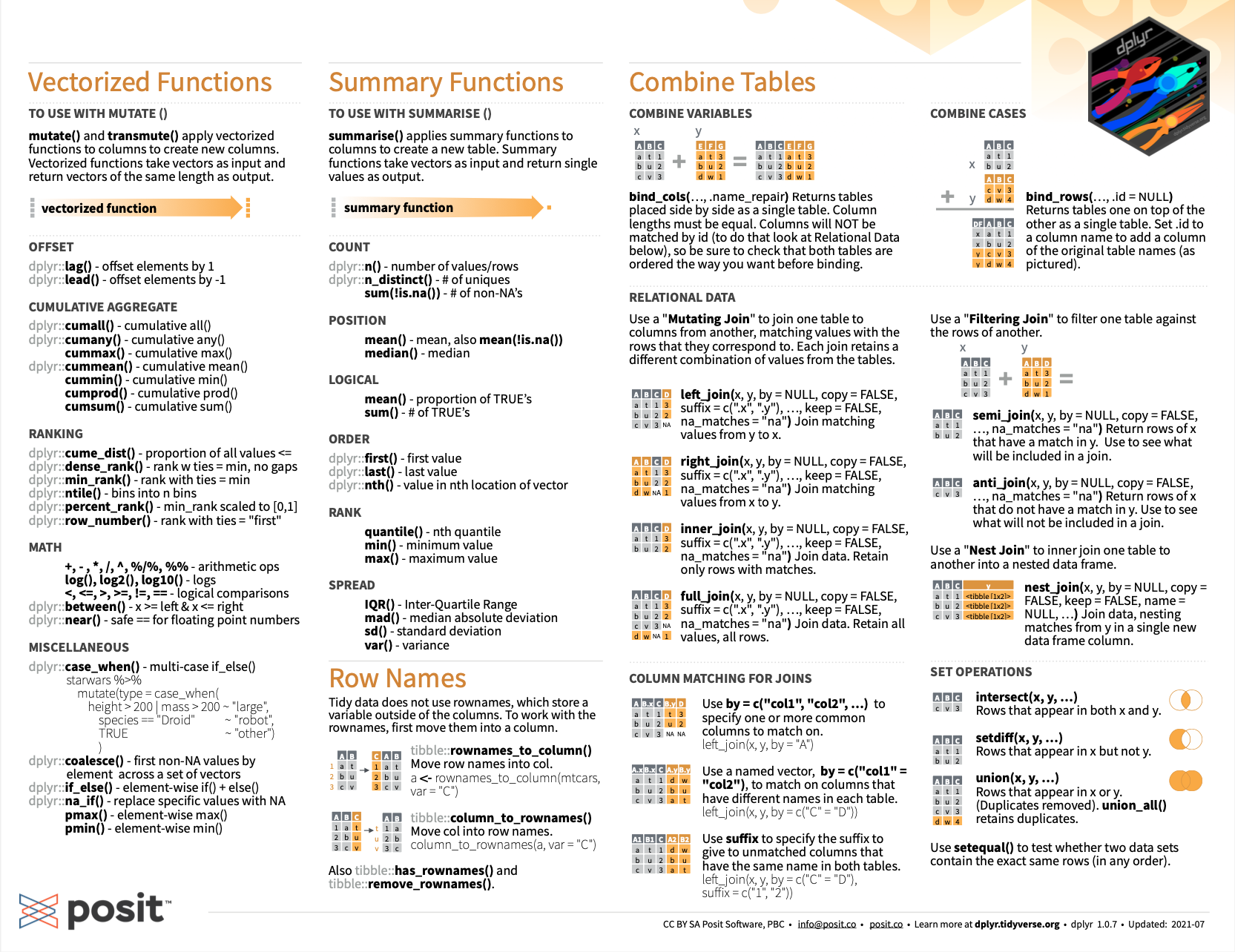## 8.3 SummaryThis chapter showed us various ways of combining two tables by using the two-table verbs of the dplyr package . Depending on the type of combination we wanted to achieve, we used various join() commands or set operations.

After working through this chapter, you should be able to use two-table verbs of dplyr to:

1. perform various mutating joins on two tables;
2. perform various filtering joins on two tables;
3. combine the cases of two tables with set operations; and
4. know that the base R function merge() also allows performing mutating joins.

The same procedure can be applied repeatedly to generalize beyond situations that only contain two tables. Take a look at the second page of the RStudio cheatsheet on Data transformation to check which commands you are now familiar with and which others you can still discover in the future:Figure 8.2: Joining data with 2-table verbs of dplyr
(summary from RStudio cheatsheets).

Let’s test our knowledge and skills on joining tables by completing the following exercises.

### References

Wickham, H., François, R., Henry, L., & Müller, K. (2022). Dplyr: A grammar of data manipulation. Retrieved from https://CRAN.R-project.org/package=dplyr